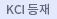#다국어 입력

http://chineseinput.net/에서 pinyin(병음)방식으로 중국어를 변환할 수 있습니다.

변환된 중국어를 복사하여 사용하시면 됩니다.

예시)
• 中文 을 입력하시려면 zhongwen을 입력하시고 space를누르시면됩니다.
• 北京 을 입력하시려면 beijing을 입력하시고 space를 누르시면 됩니다.
닫기

검색결과 좁혀 보기

선택해제
• 좁혀본 항목 보기순서

• 원문유무
• 원문제공처
• 등재정보
• 학술지명
펼치기
• 주제분류
• 발행연도
펼치기
• 작성언어
• 저자
펼치기

오늘 본 자료

• 오늘 본 자료가 없습니다.
더보기
• 무료
• 기관 내 무료
• 유료
•플라즈마 화학증착법에서 증착변수가 TiN 증착에 미치는 영향(III) -r.f. power 및 전극간 거리를 중심으로-

To investigate the influence of r.f. power and electrode distance on the TiN deposition, TiN films were deposited onto STC3, STD11 steel and Si-wafer from gas mixtures of $TiC_4/N_2/H_2$ using the radio frequency plasma assisted chemical vapor deposition. The crystallinity of TiN film could be improved by the increase of r.f. power and the decrease of electrode distance. The TiN coated layer contains chlorine, its content were decreased with increasing r.f. power as well as decreasing electrode distance. And the thickness of deposited TiN was largely affected by r.f. power and electrode distance. The hardness of deposited TiN reached a maximum value of about Hv 2,000.

• 황처리가 금속/InP Schootky 접촉과 $Si_3$$N_4$/InP 계면들에 미치는 영향

The effects of sulfur treatments on the barrier heithts of Schottky contacts and the interface-state density of metal-insulator-semiconductor (MIS) capacitors on InP have been investigated. Schottky contacts were formed by the evaporation of Al, Au, and Pt on n-InP substrate before and after (NH$_{4}$)$_{2}$S$_{x}$ treatments, respectively. The barrier height of InP Schottky contacts was measured by their current-voltage (I-V) and capacitance-voltage (C_V) characteristics. We observed that the barrier heights of Schottky contacks on bare InP were 0.35~0.45 eV nearly independent of the metal work function, which is known to be due to the surface Fermi level pinning. In the case of sulfur-treated Au/InP ar Pt/InP Schottky diodes, However, the barrier heights were not only increased above 0.7 eV but also highly dependent on the metal work function. We have also investigated effects of (NH$_{4}$)$_{2}$S$_{x}$ treatments on the distribution of interface states in Si$_{3}$N$_{4}$InP MIS diodes where Si$_{3}$N$_{4}$ was provided by plasma enhanced chemical vapor deposition (PECVD). The typical value of interface-state density extracted feom 1 MHz C-V curve of sulfur-treated SiN$_{x}$/InP MIS diodes was found to be the order of 5${\times}10^{10}cm^{2}eV^{1}$. This value is much lower than that of MiS diodes made on bare InP surface. It is certain, therefore, that the (NH$_{4}$)$_{2}$S$_{x}$ treatment is a very powerful tool to enhance the barrier heights of Au/n-InP and Pt/n-InP Schottky contacts and to reduce the density of interface states in SiN$_{x}$/InP MIS diode.

• 미세 선폭 프린팅을 위한 롤투롤 장비 개발

Printing technology has begun to get into the spotlight in many ways due to the low cost effectiveness to existent semiconductor process. It also has very useful application areas, not only paper printing but also patterning for LCD color filter, Photovoltaic patterning, RFID antenna, OLED, and so on. In this study, an apparatus of gravure offset printing was developed for fine line width printing. The pattern was composed of 20㎛ size of continuous lines of which pitch size was 40㎛. The printed pattern shows that it is possible to make around 20㎛ line-width printing pattern. The roll-to-roll printing system for fine line-width printing based on primary experiment is presented. For testing of multi-layer printing, the system was designed to be capable of printing two different materials from each printing unit using gravure-offset printing method and have a function of alignment of two printed materials.

해외이동버튼